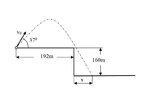# Kinematics - can't find the initial velocity according to the image

• Physics_learner
In summary, the conversation discusses how to find the initial velocity and final x position of a projectile launched from a 160m cliff at 37 degrees above the horizontal. It is suggested to use the position over time equation in the y direction and the motion equation in the x direction to solve for the two unknowns. Another approach is to split the problem into two parts, finding the initial speed first and then solving for x in the figure.

#### Physics_learner

Homework Statement
Kinematics - finding the initial velocity and final x position
Relevant Equations
x(t)=x0+v0*(t2-t1)+0.5a*(t2-2a)^2
v(t)=v0+a*(t2-t1)
I tried to write the data I understood from the image:
y0=160m
yf=0
x0=0
x1=192m
I tried to express the total change in time using the position over time equation on the Y direction:
y(t)=y0+v0y*(t2-t1)-0.5a(t2-t1)^2
but then I stuck with 2 variables and didn't know what to do

any help?

#### Attachments

•1646077929948.png
4 KB · Views: 42
Physics_learner said:
Homework Statement:: Kinematics - finding the initial velocity and final x position
Relevant Equations:: x(t)=x0+v0*(t2-t1)+0.5a*(t2-2a)^2
Welcome to PF.

It looks like you are on the right track, except you seem to have a typo in your last relevant equation (in the ##t^2## term). Can you fix that?

And you are taking the right approach, writing one equation for the motion in the y direction, and now you should add the 2nd equation which is for the motion in the x direction. Hint: the x-direction velocity does not slow down or change, if you neglect air resistance.

Then for your two equations, you use ## sin \theta ## and ## cos \theta ## appropriately to give you the components of the initial velocity in the two directions (y and x), and work on solving the two unknowns using the two equations. Does that help?

•Lnewqban
A different approach, which is perhaps conceptually easier to implement, is to split the problem in two parts. Note that when the projectile returns to the same height at the corner of the step, its speed is the same but the vertical component has changed direction and is down instead of up.

To find the initial speed ##v_0##, solve the problem "A projectile is fired at 37° above the horizontal and travels 192 m horizontally before it returns to the same height. Find the initial speed ##v_0##."

To find ##x## in the figure, solve the problem "A projectile is fired from the edge of a 160 m cliff at 37° below the horizontal. When it lands, it is horizontal distance ##x## from the edge. The initial speed is the same as in the previous problem. Find ##x##." (Don't forget that the final horizontal position is 192 + ##x~##meters.)